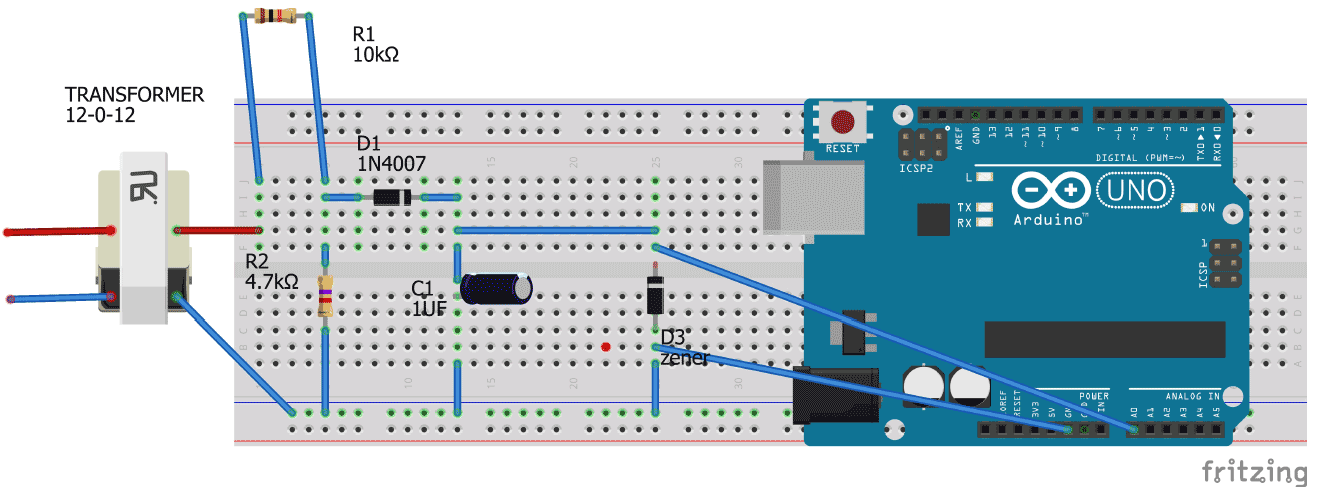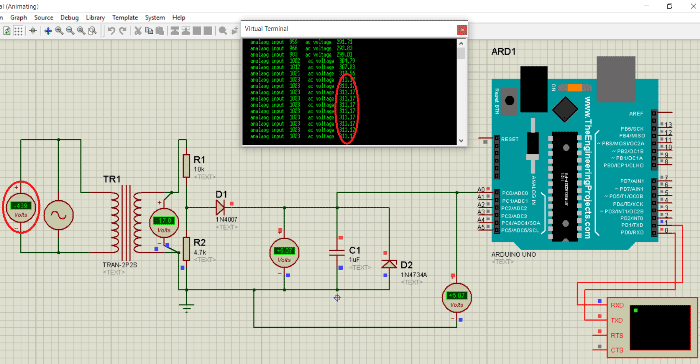# 如何使用Arduino制作一个交流电压测量设备

1336人已加入

### 所需组件：

1. 一台 12-0-12 变压器
2. 1N4007二极管
3. 1uf电容器
4. 电阻 10k;4.7k。
5. 齐纳二极管（5V）
6. Arduino UNO
7. 连接线

### Arduino电压表电路图：Arduino电压表的电路图如上所示。

1. 将变压器的高压侧（220V）连接到主电源，将低压侧（12V）连接到分压器电路。
2. 将 10k 电阻与 4.7k 电阻串联，但确保将电压作为 4.7k 电阻的输入。
3. 如图所示连接二极管。
4. 连接 4.7k 上的电容器和齐纳二极管
5. 将一根电线从二极管的n端连接到Arduino的模拟引脚A0。

** 注意：将Arduino的接地引脚连接到图中所示的点，否则电路将不起作用。### 基于Arduino的交流电压表的工作：

1. 变压器的L.V侧获得降压电压，适用于正常额定功率电阻器。

2.然后我们在4.7k电阻上得到合适的电压值

1. Arduino 将此电压作为引脚 A0 的输入，以 0 到 1023 之间的模拟值的形式出现。0 是 0 伏，1023 是 5V。
2. 然后，Arduino 通过公式将此模拟值转换为相应的电源交流电压。（在代码部分中解释）。

### 模拟：### 代码说明：

m 是引脚 A0 上接收到的输入模拟值，即

``````m=    pinMode (A0,INPUT) ;  // set pin a0 as input pin
``````

1023 模拟值对应于 311 伏电源

n=（311/1023） m 伏特或 n=（m .304177）

``````Serial.print("   analog input  ") ; // this gives name which is “analog input”  to the printed analog value

Serial.print(m);// this simply prints the input analog value
``````

``````Serial.print("   ac voltage  ") ; // this gives name  “ac voltage”  to the printed analog value

Serial.print(n) ;  // this simply prints the ac voltage value
``````

int m;// initialise variable m

float n;//initialise variable n

void setup()

{

pinMode(A0,INPUT); // set pin a0 as input pin

Serial.begin(9600);// begin serial communication between arduino and pc

}

void loop()

{

n=(m* .304177);// converts analog value(x) into input ac supply value using this formula ( explained in woeking section)

Serial.print(" analaog input " ) ; // specify name to the corresponding value to be printed

Serial.print(m) ; // print input analog value on serial monitor

Serial.print(" ac voltage ") ; // specify name to the corresponding value to be printed

Serial.print(n) ; // prints the ac value on Serial monitor

Serial.println();

}

``````
``````×
20